## Quiz 7 : Speculation and Risk in the Foreign Exchange MarketLooking for Finance Homework Help?# Quiz 7 : Speculation and Risk in the Foreign Exchange Market

Current spot exchange rate of USD and GBP are provided with the probability of change in the exchange rate in order to predict the future spot exchange rate.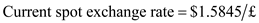As per the given information, all the probable change is equally likely to happen. In this case excel can be used. Below excel discloses the data entered for the calculation purposes: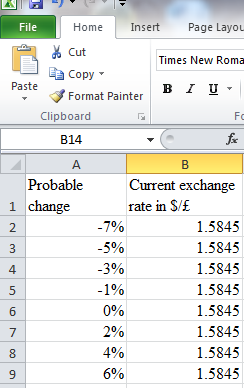First the probability of occurrence has to be determined. There are 8 probabilities which indicates that there are 1/8 chances for each and every probability to occur. Enter in excel C2 to C9 "=1/8" to determine the probability. Below excel discloses the formula used: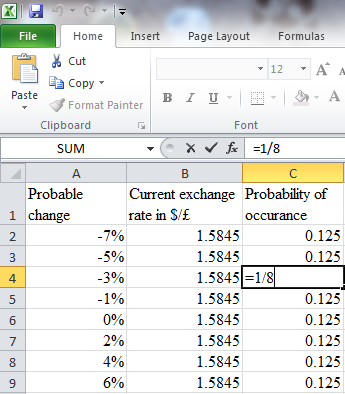This can be verified by entering in B10 "=SUM(C2:C9)" to determine the total probability. Below excel discloses the formula entered: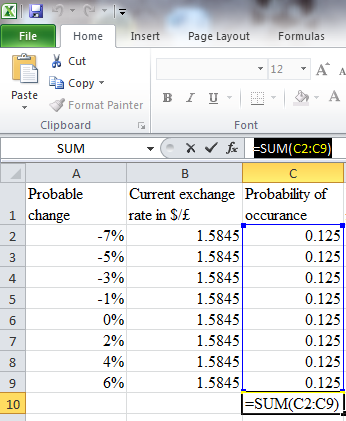Probability table: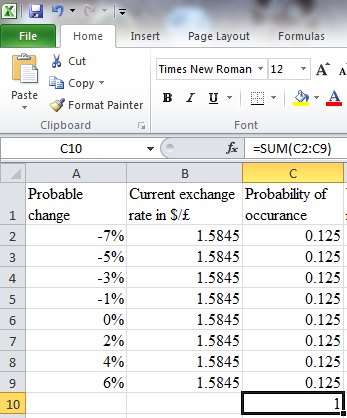Enter in excel D2 "=B2*(1+A2)" to D9 "=B9*(1+A9)" for determining the currency value with the respective change. Below excel discloses the formula entered: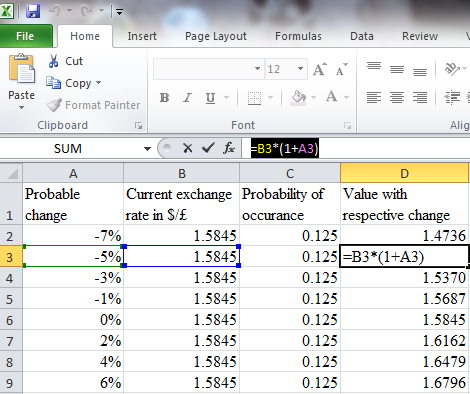Enter in excel D10 "=AVERAGE(D2:D9)" to determine the mean currency rate. Below excel discloses the mean currency value: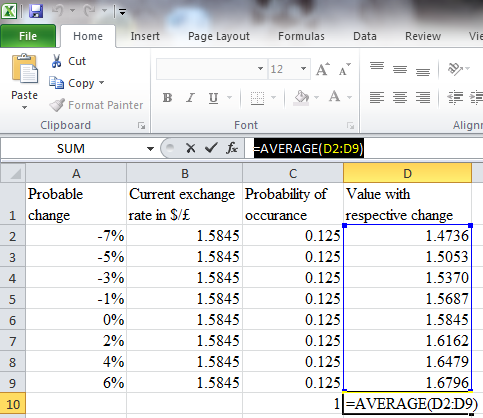Mean currency value: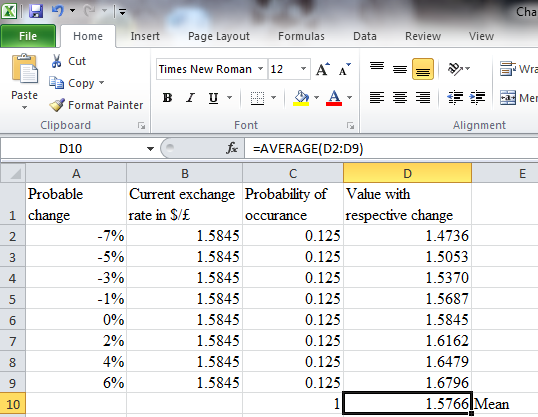Enter in excel D11 "=STDEVP(D2:D10)" to determine the standard deviation currency rate. Below excel discloses the mean currency value: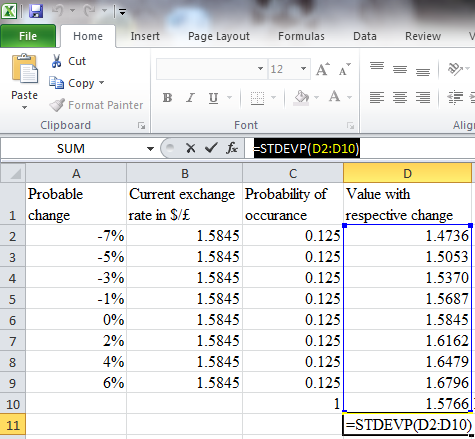Standard deviation: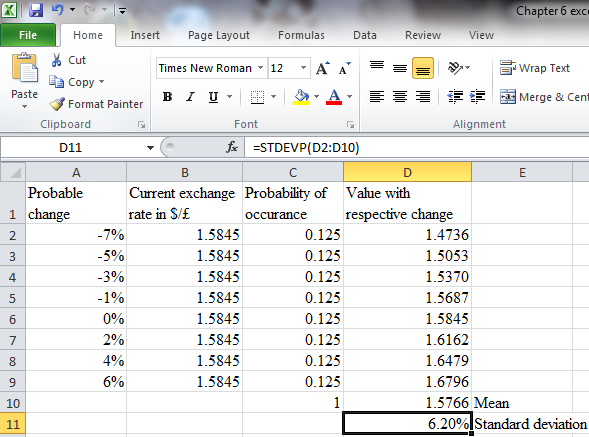Thus, the mean is 1.5766 and Standard deviation is 6.20%.

The speculator should borrow in that currency which is expected to weaken in the future and invest borrowed amount in those currencies which is expected to be appreciated. If the currency movement is as per the expectation, then the speculator will reap the benefit from the investment including the interest from the strengthened currency. At expiry date the maturity receipts will be converted into weaker currency to repay the borrowings, thus the speculator made profit without any upfront investment. Alternatively, the speculator enters into a forward contract to buy a currency which is expected to strengthen in the future. In this case the speculator will buy the currency at the agreed forward rate and will sell at the current higher spot rate, and this transaction will results in more profit. Similarly, the speculator can sell the currency in forward market which is expected to weaken and book profit by selling at higher price and buying at lower spot rate. The above mentioned are the methods where the speculator is not required to make upfront investment for booking profits. On the other hand, a speculator will gain only if the expectations are correct, any adverse movement than the expected movement will result in loss to the speculators.

From the given information, it is clear that the peso has depreciated and is expected to depreciate further. The peso recently lost over 40% of its value relative to the dollar. Over the course of the next 90 days, the Mexican government risks losing control of the economy.  In Case 1 The probability of losing the control of economy is 35% (0.35). There is a fall in Mexican peso of 33%. Fall in stock market is 39%. Hence, \$1 invested in the stock market will become \$0.61 in case 1. In case 2 The probability of losing the control of economy is 65% (0.65). There is a rise in Mexican peso of 27%. Rise in stock market is 29%. Hence, \$1 invested in the stock market will become \$1.29 in case 2. Therefore, taking both cases together, \$1 investment in stock market will become: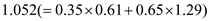Hence, the return from stock market will be 5.2%. Calculate the absolute appreciation/depreciation of peso: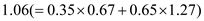Therefore, the investors return in Dollar will be:Hence, expected dollar return from investing dollars in the Mexican stock market is 4.90%.

There is no answer for this question

There is no answer for this question